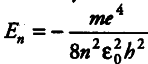# Positronium is just like a H-atom with the proton

Positronium is just like a H-atom with the proton replaced by the positively charged anti-particle of the electron (called the positron which is as massive as the electron). What would be the ground state energy of positronium?

The total energy of the electron in the stationary states of the hydrogen atom is given bywhere signs are as usual and the m that occurs in the Bohr formula is the reduced mass of electron and proton. Also, the total energy of the electron in the ground state of the hydrogen atom is -13.6 eV. For H-atom, reduced mass is { m }_{ e } whereas the positornium , the reduced mass is
m = { m }_{ e } / 2
Hence, the total energy of the electron in the ground state of the positronium atom is -13.6eV / 2 = -6.8 eV.